Uniform Circular Motion and Gravitation

# Centripetal Force

OpenStaxCollege

### Learning Objectives

• Calculate coefficient of friction on a car tire.
• Calculate ideal speed and angle of a car on a turn.

Any force or combination of forces can cause a centripetal or radial acceleration. Just a few examples are the tension in the rope on a tether ball, the force of Earth’s gravity on the Moon, friction between roller skates and a rink floor, a banked roadway’s force on a car, and forces on the tube of a spinning centrifuge.

Any net force causing uniform circular motion is called a centripetal force. The direction of a centripetal force is toward the center of curvature, the same as the direction of centripetal acceleration. According to Newton’s second law of motion, net force is mass times acceleration: net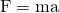. For uniform circular motion, the acceleration is the centripetal acceleration—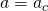. Thus, the magnitude of centripetal force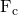is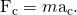By using the expressions for centripetal acceleration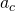from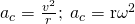, we get two expressions for the centripetal forcein terms of mass, velocity, angular velocity, and radius of curvature: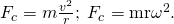You may use whichever expression for centripetal force is more convenient. Centripetal force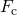is always perpendicular to the path and pointing to the center of curvature, because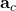is perpendicular to the velocity and pointing to the center of curvature.

Note that if you solve the first expression for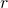, you get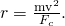This implies that for a given mass and velocity, a large centripetal force causes a small radius of curvature—that is, a tight curve.

The frictional force supplies the centripetal force and is numerically equal to it. Centripetal force is perpendicular to velocity and causes uniform circular motion. The larger the, the smaller the radius of curvatureand the sharper the curve. The second curve has the same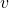, but a largerproduces a smaller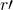.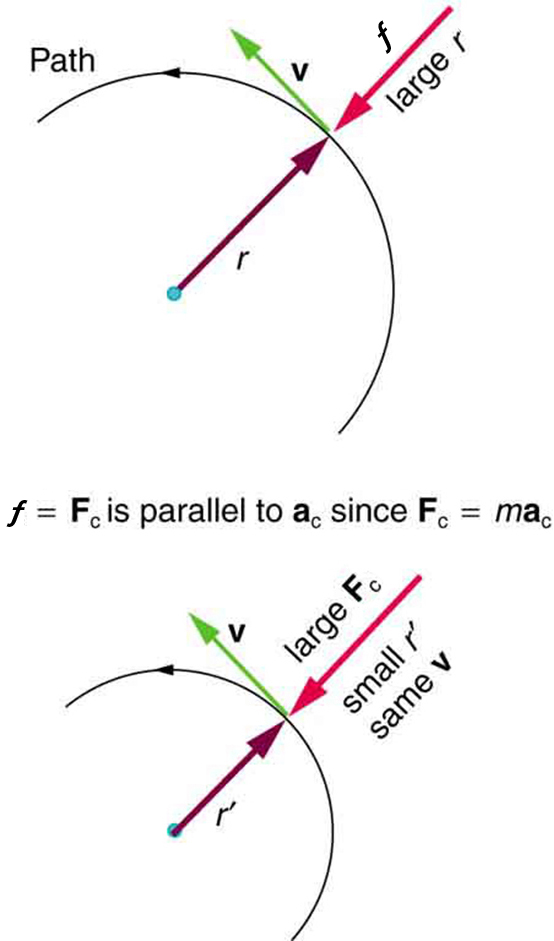What Coefficient of Friction Do Car Tires Need on a Flat Curve?

(a) Calculate the centripetal force exerted on a 900 kg car that negotiates a 500 m radius curve at 25.0 m/s.

(b) Assuming an unbanked curve, find the minimum static coefficient of friction, between the tires and the road, static friction being the reason that keeps the car from slipping (see [link]).

Strategy and Solution for (a)

We know that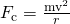. Thus,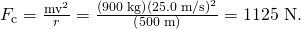Strategy for (b)

[link] shows the forces acting on the car on an unbanked (level ground) curve. Friction is to the left, keeping the car from slipping, and because it is the only horizontal force acting on the car, the friction is the centripetal force in this case. We know that the maximum static friction (at which the tires roll but do not slip) is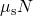, where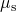is the static coefficient of friction and N is the normal force. The normal force equals the car’s weight on level ground, so that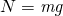. Thus the centripetal force in this situation is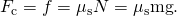Now we have a relationship between centripetal force and the coefficient of friction. Using the first expression forfrom the equation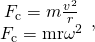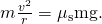We solve this for, noting that mass cancels, and obtain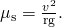Solution for (b)

Substituting the knowns,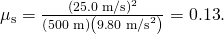(Because coefficients of friction are approximate, the answer is given to only two digits.)

Discussion

We could also solve part (a) using the first expression inbecause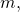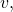andare given. The coefficient of friction found in part (b) is much smaller than is typically found between tires and roads. The car will still negotiate the curve if the coefficient is greater than 0.13, because static friction is a responsive force, being able to assume a value less than but no more than. A higher coefficient would also allow the car to negotiate the curve at a higher speed, but if the coefficient of friction is less, the safe speed would be less than 25 m/s. Note that mass cancels, implying that in this example, it does not matter how heavily loaded the car is to negotiate the turn. Mass cancels because friction is assumed proportional to the normal force, which in turn is proportional to mass. If the surface of the road were banked, the normal force would be less as will be discussed below.

This car on level ground is moving away and turning to the left. The centripetal force causing the car to turn in a circular path is due to friction between the tires and the road. A minimum coefficient of friction is needed, or the car will move in a larger-radius curve and leave the roadway.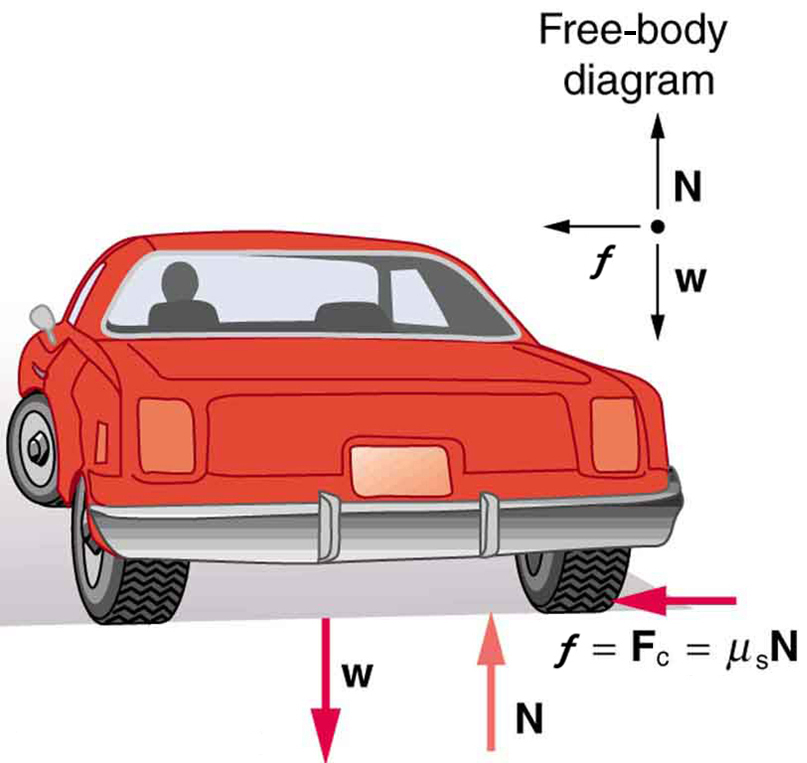Let us now consider banked curves, where the slope of the road helps you negotiate the curve. See [link]. The greater the angle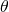, the faster you can take the curve. Race tracks for bikes as well as cars, for example, often have steeply banked curves. In an “ideally banked curve,” the angleis such that you can negotiate the curve at a certain speed without the aid of friction between the tires and the road. We will derive an expression forfor an ideally banked curve and consider an example related to it.

For ideal banking, the net external force equals the horizontal centripetal force in the absence of friction. The components of the normal force N in the horizontal and vertical directions must equal the centripetal force and the weight of the car, respectively. In cases in which forces are not parallel, it is most convenient to consider components along perpendicular axes—in this case, the vertical and horizontal directions.

[link] shows a free body diagram for a car on a frictionless banked curve. If the angleis ideal for the speed and radius, then the net external force will equal the necessary centripetal force. The only two external forces acting on the car are its weight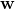and the normal force of the road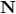. (A frictionless surface can only exert a force perpendicular to the surface—that is, a normal force.) These two forces must add to give a net external force that is horizontal toward the center of curvature and has magnitude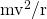. Because this is the crucial force and it is horizontal, we use a coordinate system with vertical and horizontal axes. Only the normal force has a horizontal component, and so this must equal the centripetal force—that is,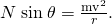Because the car does not leave the surface of the road, the net vertical force must be zero, meaning that the vertical components of the two external forces must be equal in magnitude and opposite in direction. From the figure, we see that the vertical component of the normal force is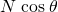, and the only other vertical force is the car’s weight. These must be equal in magnitude; thus,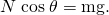Now we can combine the last two equations to eliminate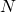and get an expression for, as desired. Solving the second equation for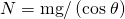, and substituting this into the first yields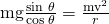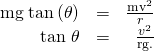Taking the inverse tangent gives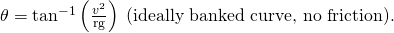This expression can be understood by considering howdepends onand. A largewill be obtained for a largeand a small. That is, roads must be steeply banked for high speeds and sharp curves. Friction helps, because it allows you to take the curve at greater or lower speed than if the curve is frictionless. Note thatdoes not depend on the mass of the vehicle.

The car on this banked curve is moving away and turning to the left.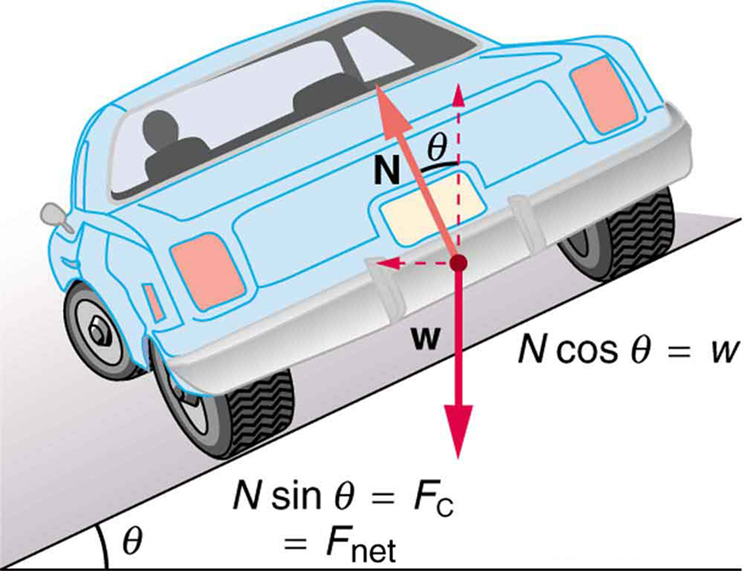What Is the Ideal Speed to Take a Steeply Banked Tight Curve?

Curves on some test tracks and race courses, such as the Daytona International Speedway in Florida, are very steeply banked. This banking, with the aid of tire friction and very stable car configurations, allows the curves to be taken at very high speed. To illustrate, calculate the speed at which a 100 m radius curve banked at 65.0° should be driven if the road is frictionless.

Strategy

We first note that all terms in the expression for the ideal angle of a banked curve except for speed are known; thus, we need only rearrange it so that speed appears on the left-hand side and then substitute known quantities.

Solution

Starting with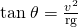we get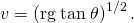Noting that tan 65.0º = 2.14, we obtain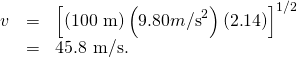Discussion

This is just about 165 km/h, consistent with a very steeply banked and rather sharp curve. Tire friction enables a vehicle to take the curve at significantly higher speeds.

Calculations similar to those in the preceding examples can be performed for a host of interesting situations in which centripetal force is involved—a number of these are presented in this chapter’s Problems and Exercises.

Take-Home Experiment

Ask a friend or relative to swing a golf club or a tennis racquet. Take appropriate measurements to estimate the centripetal acceleration of the end of the club or racquet. You may choose to do this in slow motion.

PhET Explorations: Gravity and Orbits

Move the sun, earth, moon and space station to see how it affects their gravitational forces and orbital paths. Visualize the sizes and distances between different heavenly bodies, and turn off gravity to see what would happen without it!

# Section Summary

• Centripetal forceis any force causing uniform circular motion. It is a “center-seeking” force that always points toward the center of rotation. It is perpendicular to linear velocityand has magnitude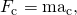which can also be expressed as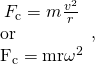# Conceptual Questions

If you wish to reduce the stress (which is related to centripetal force) on high-speed tires, would you use large- or small-diameter tires? Explain.

Define centripetal force. Can any type of force (for example, tension, gravitational force, friction, and so on) be a centripetal force? Can any combination of forces be a centripetal force?

If centripetal force is directed toward the center, why do you feel that you are ‘thrown’ away from the center as a car goes around a curve? Explain.

Race car drivers routinely cut corners as shown in [link]. Explain how this allows the curve to be taken at the greatest speed.

Two paths around a race track curve are shown. Race car drivers will take the inside path (called cutting the corner) whenever possible because it allows them to take the curve at the highest speed.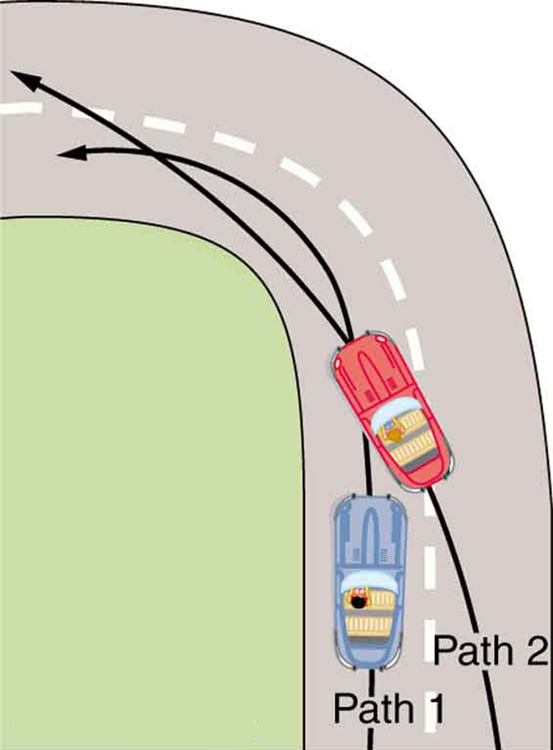A number of amusement parks have rides that make vertical loops like the one shown in [link]. For safety, the cars are attached to the rails in such a way that they cannot fall off. If the car goes over the top at just the right speed, gravity alone will supply the centripetal force. What other force acts and what is its direction if:

(a) The car goes over the top at faster than this speed?

(b)The car goes over the top at slower than this speed?

Amusement rides with a vertical loop are an example of a form of curved motion.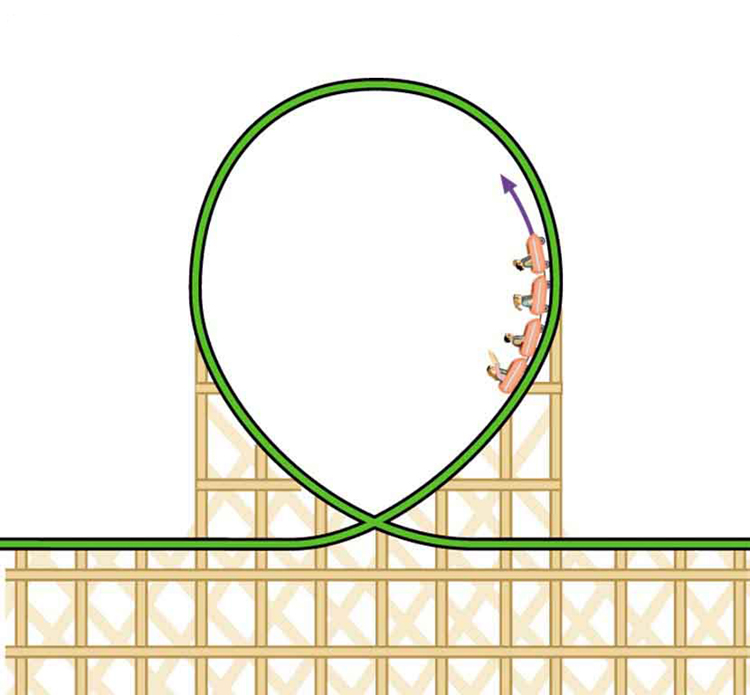What is the direction of the force exerted by the car on the passenger as the car goes over the top of the amusement ride pictured in [link] under the following circumstances:

(a) The car goes over the top at such a speed that the gravitational force is the only force acting?

(b) The car goes over the top faster than this speed?

(c) The car goes over the top slower than this speed?

As a skater forms a circle, what force is responsible for making her turn? Use a free body diagram in your answer.

Suppose a child is riding on a merry-go-round at a distance about halfway between its center and edge. She has a lunch box resting on wax paper, so that there is very little friction between it and the merry-go-round. Which path shown in [link] will the lunch box take when she lets go? The lunch box leaves a trail in the dust on the merry-go-round. Is that trail straight, curved to the left, or curved to the right? Explain your answer.

A child riding on a merry-go-round releases her lunch box at point P. This is a view from above the clockwise rotation. Assuming it slides with negligible friction, will it follow path A, B, or C, as viewed from Earth’s frame of reference? What will be the shape of the path it leaves in the dust on the merry-go-round?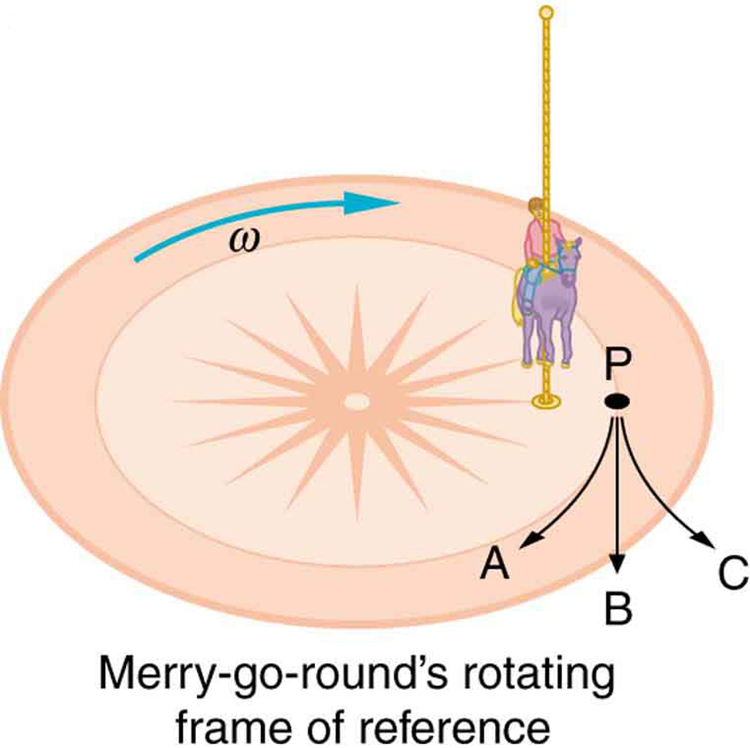Do you feel yourself thrown to either side when you negotiate a curve that is ideally banked for your car’s speed? What is the direction of the force exerted on you by the car seat?

Suppose a mass is moving in a circular path on a frictionless table as shown in figure. In the Earth’s frame of reference, there is no centrifugal force pulling the mass away from the centre of rotation, yet there is a very real force stretching the string attaching the mass to the nail. Using concepts related to centripetal force and Newton’s third law, explain what force stretches the string, identifying its physical origin.

A mass attached to a nail on a frictionless table moves in a circular path. The force stretching the string is real and not fictional. What is the physical origin of the force on the string?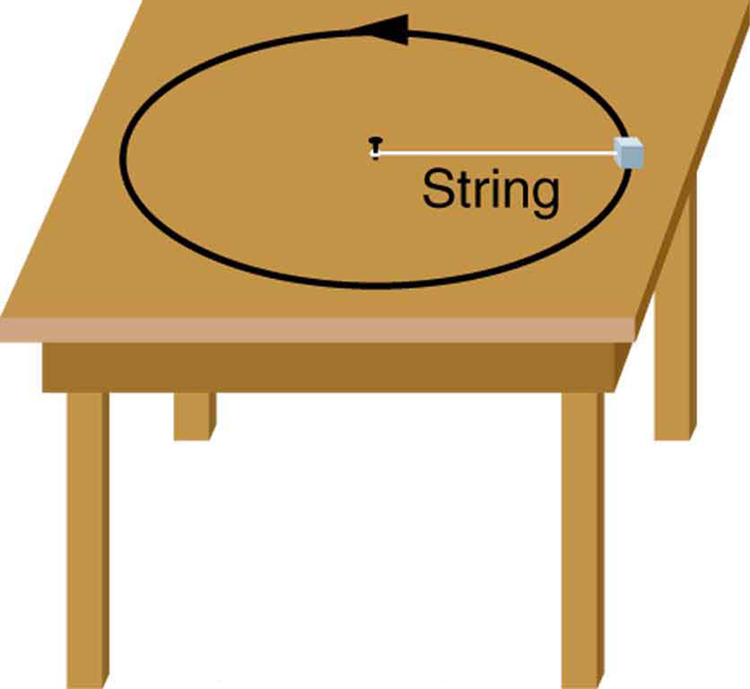# Problems Exercise

(a) A 22.0 kg child is riding a playground merry-go-round that is rotating at 40.0 rev/min. What centripetal force must she exert to stay on if she is 1.25 m from its center?

(b) What centripetal force does she need to stay on an amusement park merry-go-round that rotates at 3.00 rev/min if she is 8.00 m from its center?

(c) Compare each force with her weight.

a) 483 N

b) 17.4 N

c) 2.24 times her weight, 0.0807 times her weight

Calculate the centripetal force on the end of a 100 m (radius) wind turbine blade that is rotating at 0.5 rev/s. Assume the mass is 4 kg.

What is the ideal banking angle for a gentle turn of 1.20 km radius on a highway with a 105 km/h speed limit (about 65 mi/h), assuming everyone travels at the limit?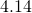What is the ideal speed to take a 100 m radius curve banked at a 20.0° angle?

(a) What is the radius of a bobsled turn banked at 75.0° and taken at 30.0 m/s, assuming it is ideally banked?

(b) Calculate the centripetal acceleration.

(c) Does this acceleration seem large to you?

a) 24.6 m

b)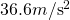c)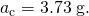This does not seem too large, but it is clear that bobsledders feel a lot of force on them going through sharply banked turns.

Part of riding a bicycle involves leaning at the correct angle when making a turn, as seen in [link]. To be stable, the force exerted by the ground must be on a line going through the center of gravity. The force on the bicycle wheel can be resolved into two perpendicular components—friction parallel to the road (this must supply the centripetal force), and the vertical normal force (which must equal the system’s weight).

(a) Show that(as defined in the figure) is related to the speedand radius of curvatureof the turn in the same way as for an ideally banked roadway—that is,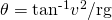(b) Calculatefor a 12.0 m/s turn of radius 30.0 m (as in a race).

A bicyclist negotiating a turn on level ground must lean at the correct angle—the ability to do this becomes instinctive. The force of the ground on the wheel needs to be on a line through the center of gravity. The net external force on the system is the centripetal force. The vertical component of the force on the wheel cancels the weight of the system while its horizontal component must supply the centripetal force. This process produces a relationship among the angle, the speed, and the radius of curvatureof the turn similar to that for the ideal banking of roadways.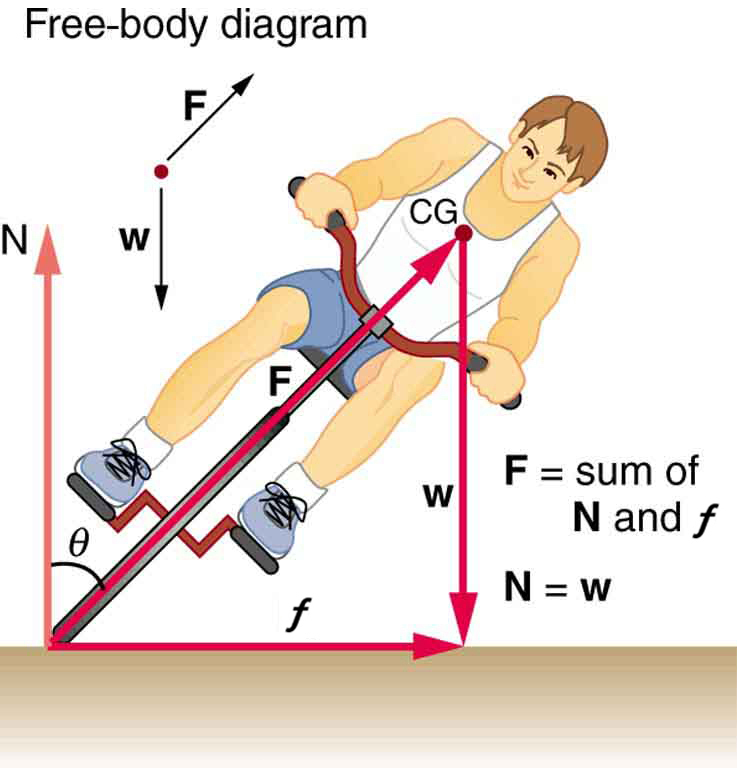A large centrifuge, like the one shown in [link](a), is used to expose aspiring astronauts to accelerations similar to those experienced in rocket launches and atmospheric reentries.

(a) At what angular velocity is the centripetal acceleration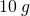if the rider is 15.0 m from the center of rotation?

(b) The rider’s cage hangs on a pivot at the end of the arm, allowing it to swing outward during rotation as shown in [link](b). At what anglebelow the horizontal will the cage hang when the centripetal acceleration is? (Hint: The arm supplies centripetal force and supports the weight of the cage. Draw a free body diagram of the forces to see what the angleshould be.)

(a) NASA centrifuge used to subject trainees to accelerations similar to those experienced in rocket launches and reentries. (credit: NASA) (b) Rider in cage showing how the cage pivots outward during rotation. This allows the total force exerted on the rider by the cage to be along its axis at all times.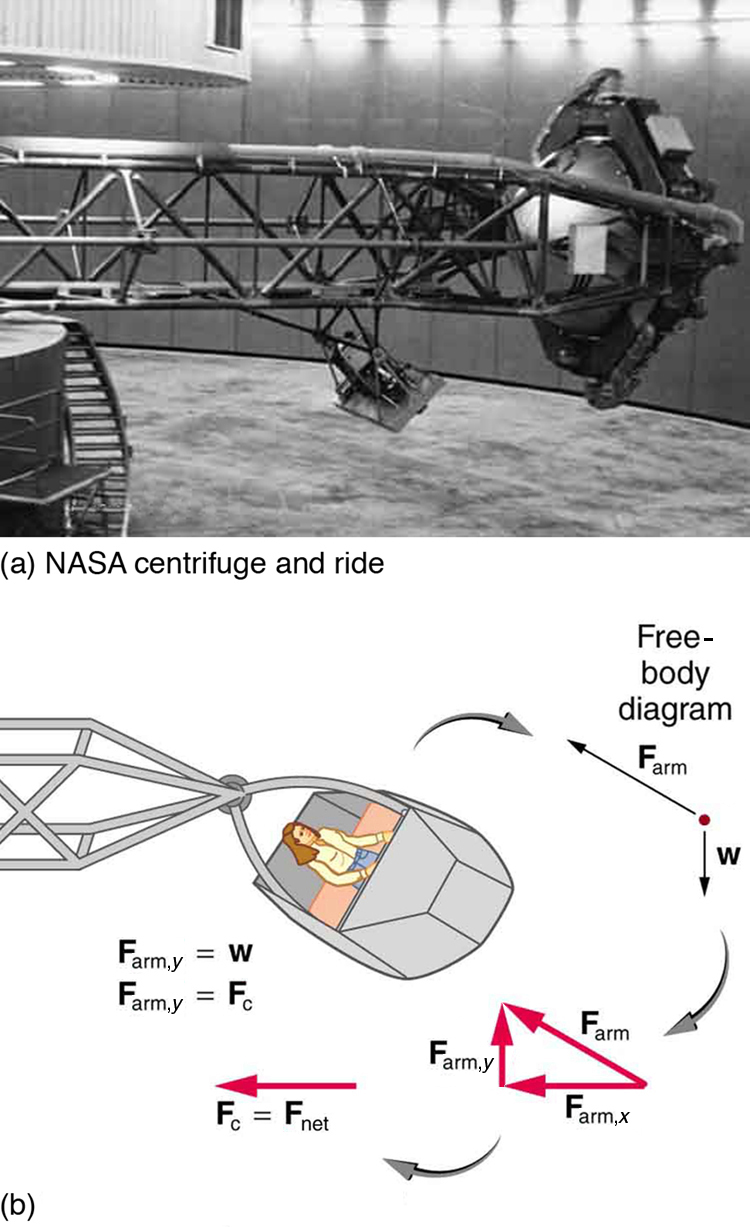b)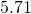Integrated Concepts

If a car takes a banked curve at less than the ideal speed, friction is needed to keep it from sliding toward the inside of the curve (a real problem on icy mountain roads). (a) Calculate the ideal speed to take a 100 m radius curve banked at 15.0º. (b) What is the minimum coefficient of friction needed for a frightened driver to take the same curve at 20.0 km/h?

a) 16.2 m/s

b) 0.234

Modern roller coasters have vertical loops like the one shown in [link]. The radius of curvature is smaller at the top than on the sides so that the downward centripetal acceleration at the top will be greater than the acceleration due to gravity, keeping the passengers pressed firmly into their seats. What is the speed of the roller coaster at the top of the loop if the radius of curvature there is 15.0 m and the downward acceleration of the car is 1.50 g?

Teardrop-shaped loops are used in the latest roller coasters so that the radius of curvature gradually decreases to a minimum at the top. This means that the centripetal acceleration builds from zero to a maximum at the top and gradually decreases again. A circular loop would cause a jolting change in acceleration at entry, a disadvantage discovered long ago in railroad curve design. With a small radius of curvature at the top, the centripetal acceleration can more easily be kept greater than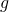so that the passengers do not lose contact with their seats nor do they need seat belts to keep them in place.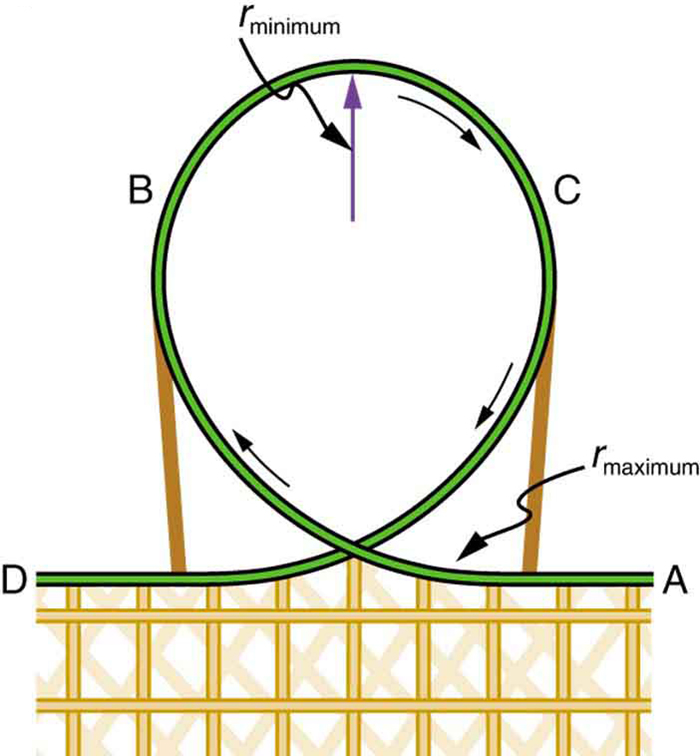Unreasonable Results

(a) Calculate the minimum coefficient of friction needed for a car to negotiate an unbanked 50.0 m radius curve at 30.0 m/s.

(b) What is unreasonable about the result?

(c) Which premises are unreasonable or inconsistent?

a) 1.84

b) A coefficient of friction this much greater than 1 is unreasonable .

c) The assumed speed is too great for the tight curve.

## Glossary

centripetal force
any net force causing uniform circular motion
ideal banking
the sloping of a curve in a road, where the angle of the slope allows the vehicle to negotiate the curve at a certain speed without the aid of friction between the tires and the road; the net external force on the vehicle equals the horizontal centripetal force in the absence of friction
ideal speed
the maximum safe speed at which a vehicle can turn on a curve without the aid of friction between the tire and the road
ideal angle
the angle at which a car can turn safely on a steep curve, which is in proportion to the ideal speed
banked curve
the curve in a road that is sloping in a manner that helps a vehicle negotiate the curve